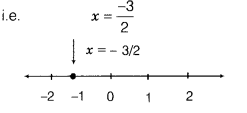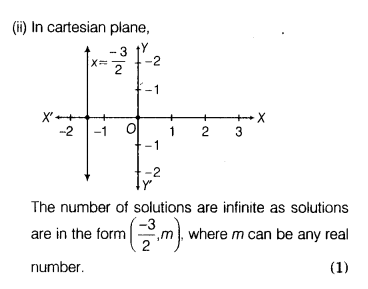# Ow many solution(s) of the equation 2(x + y) +3 =2y

How many solution(s) of the equation 2(x + y) +3 =2y are there on the
(i) number line. (ii) cartesian plane? Also, show it by the graph.

Given equation is 2(x + y) + 3 = 2ywhich is a linear equation in one variable.
(i) On number line, the solution of the given equation is unique (one solution).